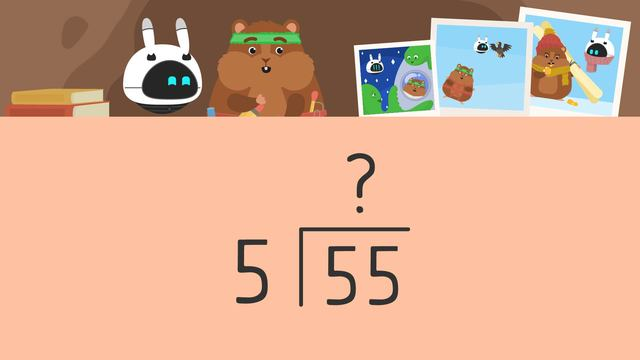# Long Division -2 Digit by 1 DigitRating

Ø 5.0 / 1 ratings

The authorsTeam Digital

## Basics on the topicLong Division -2 Digit by 1 Digit

### Content

####Long Division 2 Digit

#### Steps to Long Division 2 Digit by 1 Digit No Remainders

What are the 5 steps of long division?

#### 2 Digit Long Division Worksheets

Following the video, there is additional long division practice with

### TranscriptLong Division -2 Digit by 1 Digit

Mr. Squeaks and Imani are organizing photos of their past adventures into a photo album. They want to determine how many pages of the photo album they need for each group of photos to make sure they will all fit. We can help organize the photo album with… “ Long Division - Two Digit by One Digit" A division sentence has three parts. The dividend... the divisor... and the quotient. In long division, we organize them like THIS. The dividend is INSIDE the division bar, (...) the divisor HERE, (...) and the quotient is written on TOP. Using the pictures from Mr. Squeaks and Imani’s ski trip, we’ll use long division to calculate how to group photos in the album. They have fifty-five photos, so we’ll put that dividend HERE. They are going to put five photos per page, so that divisor will go HERE. To solve for the quotient we will follow these steps… Divide(...), multiply(...)subtract (...)bring down (...) repeat First, we DIVIDE the tens in the dividend by the divisor. To divide, we ask, how many groups of five are in five? There is ONE and we write it above the TENS place. Next, we MULTIPLY this number by the divisor... five times one equals five... and write this answer BELOW the tens in the dividend. Now, we need to SUBTRACT the numbers in the tens place. Five minus five is zero. Finally, BRING DOWN the number from the ones place... and we will repeat these steps. Five goes into five one time... and we write it HERE. Multiply one by the divisor, five. One times five is five and(...)we write that number HERE. SUBTRACT the numbers in the ones place. Five minus five is zero. There are no more numbers to bring down... so the number at the top is our quotient. Fifty-five divided by five equals eleven, so... the ski trip photos take up eleven pages of the album. Next, they have eighty-four photos from their infamous skydiving adventure, (...)and they are going to group them by sevens. We set up the problem like THIS. First, we DIVIDE seven into eight. There is ONE group of seven in eight... so, we write the one ABOVE the tens place in the quotient. Now, multiply one by the seven (...) to get seven. Where do we put the seven? (...) We put it UNDER the eight in the tens place. Now subtract,(...) eight minus seven is one. What is the next step? (...) We need to bring down the four... and repeat the steps. We now have fourteen... Seven goes into fourteen,(...) two times. Where do we write the answer? (...) Above the ones place in the quotient. What is our next step? (...) Multiply (...) two times seven equals fourteen. Where do we put our answer? (...) Under the fourteen. What is the final step? (...) Subtract(...) fourteen minus fourteen equals zero. Since there are no more numbers to bring down from the dividend, (...) this is our quotient. The skydiving photos will be on twelve pages of the album. Finally, look at the pictures from the space mission. Since there are ninety-six pictures, they are going to group them into eights. Pause the video and try this one on your own before we review the answer. How many pages do they need for the space photos? Ninety-six divided by eight is... twelve. HERE'S our work. The space photos will take up the last twelve pages of the album. Remember... long division is a way to organize the calculation of a division problem. To divide we follow these steps: Divide Multiply Subtract Bring down next place value in the dividend. Repeat steps until all digits in the dividend have been used. What’s this? (...) end after he puts together the album, we see the pics that didn't make it.

Seems like there are some photos that DIDN'T make it into the album!